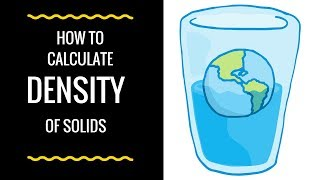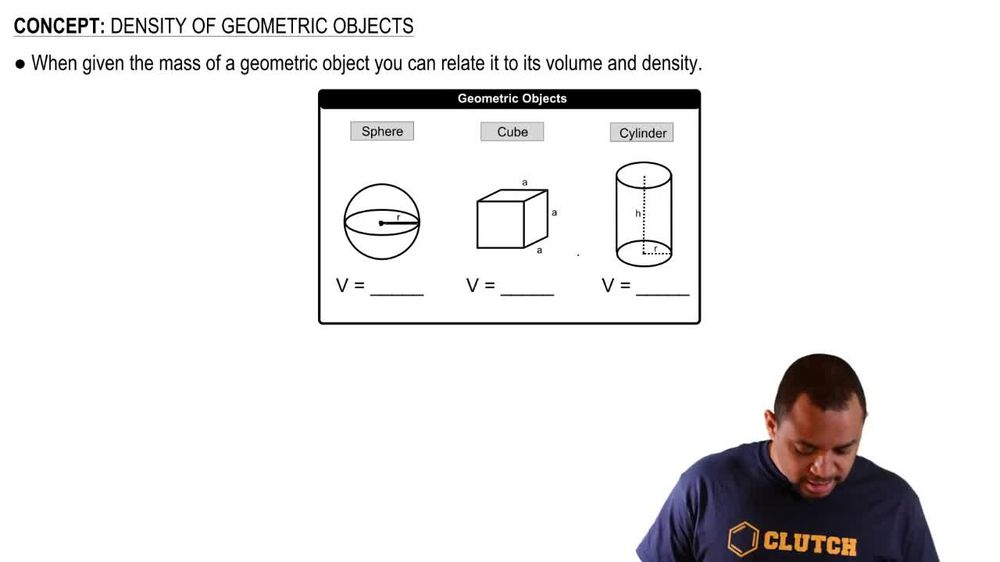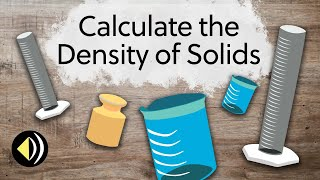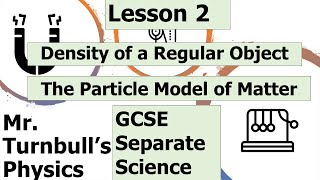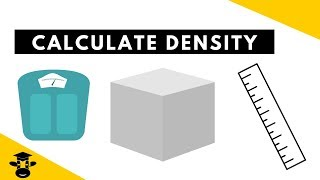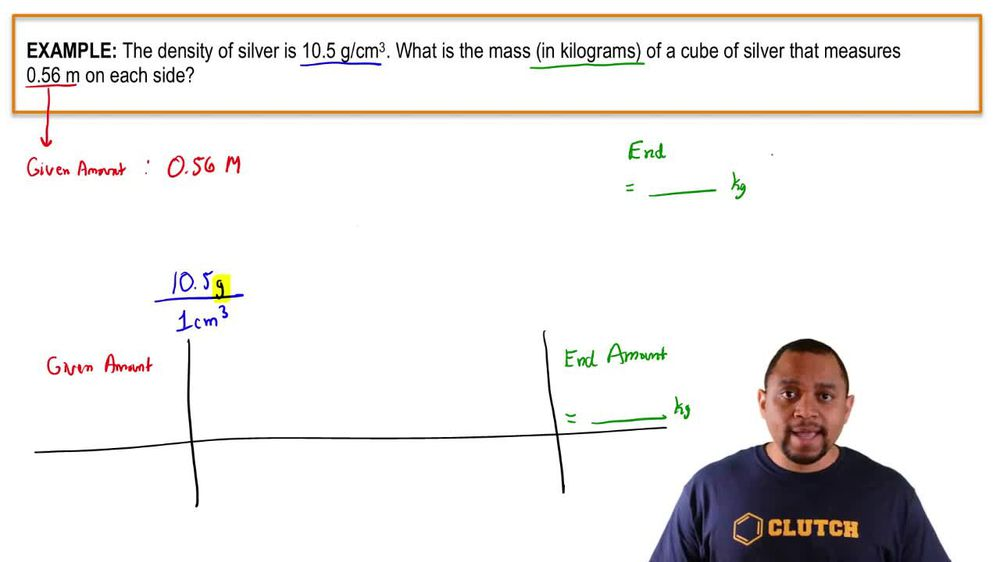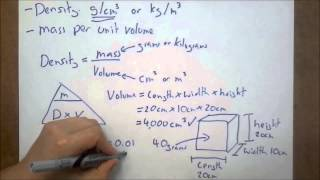Start typing, then use the up and down arrows to select an option from the list.
1. 1. Intro to General Chemistry2. Density of Geometric Objects
Problem

# The single proton that forms the nucleus of the hydrogen atom has a radius of approximately 1.0 * 10 - 13 cm. The hydrogen atom itself has a radius of approximately 52.9 pm. What fraction of the space within the atom is occupied by the nucleus?

Relevant Solution2m
Play a video:
Hello everyone today. We are being given the following problem and asked to solve for it. It says the atom. Iron 56 has 26 protons and 30 neutrons. Its nucleus has a radius of about 4.6 km toe meters. While its atomic radius is around 156 PICO meters calculate the percentage of the volume of the iron atom that is occupied by the nucleus. So the first thing we can do is we can assume that this is a perfect sphere and so in doing so we can calculate the volume for the atom. So the volume of the sphere is four thirds times pi times r cubed. We then have to have our our in terms of meters. So we'll sit at the radius of the nucleus is equal to 4.6 p. M. To meters that we have in the problem. And use the conversion factor that for every one kilometer we have one times 10 to the negative 15th meters. We can then use another conversion factor that for one PICO meter we have one times 10 to the negative 12 m. Our units are going to cancel out and we are going to be left with 4. times 10 to the negative 3rd m. We can employ our values in to the equation. So we have the volume of sphere as you go to four thirds pi and we now have our radius which is 4.6 times to the negative third meters, cute which gives us 4.772 times 10 to the negative seven PK meters cubed. Now, the volume of the atom itself is going to be the same principle with our 4/3 pi with our radius of 1 56 PK meters. Instead giving us we're gonna cube that, giving us 1.6 times 10 to the seventh meters cubed to find the percentage of the volume of the nucleus. We simply have to take our volume of the sphere 4.7 times 10 to the negative seventh and divide that by the volume of our atom, giving us 2.6 times 10 to the -12%. A very small percentage, but a percentage nonetheless, I hope this helped until next time.